Ex 2.2

Chapter 2 Class 11 Relations and Functions
Serial order wise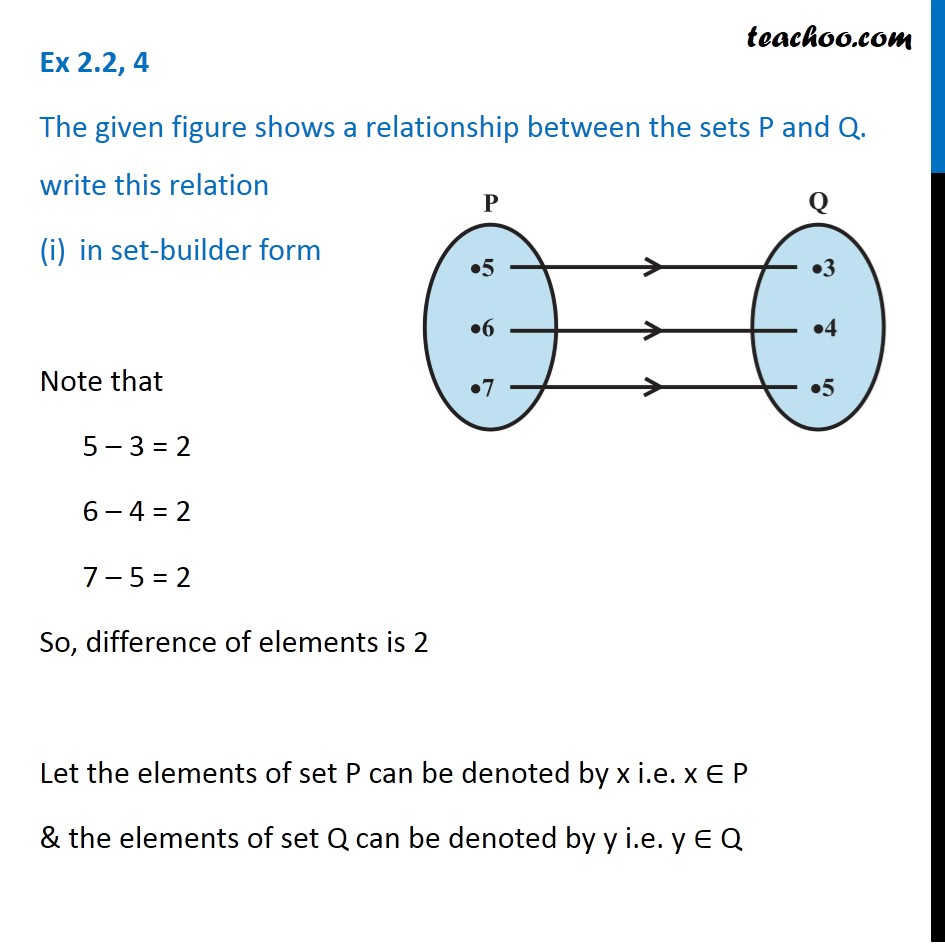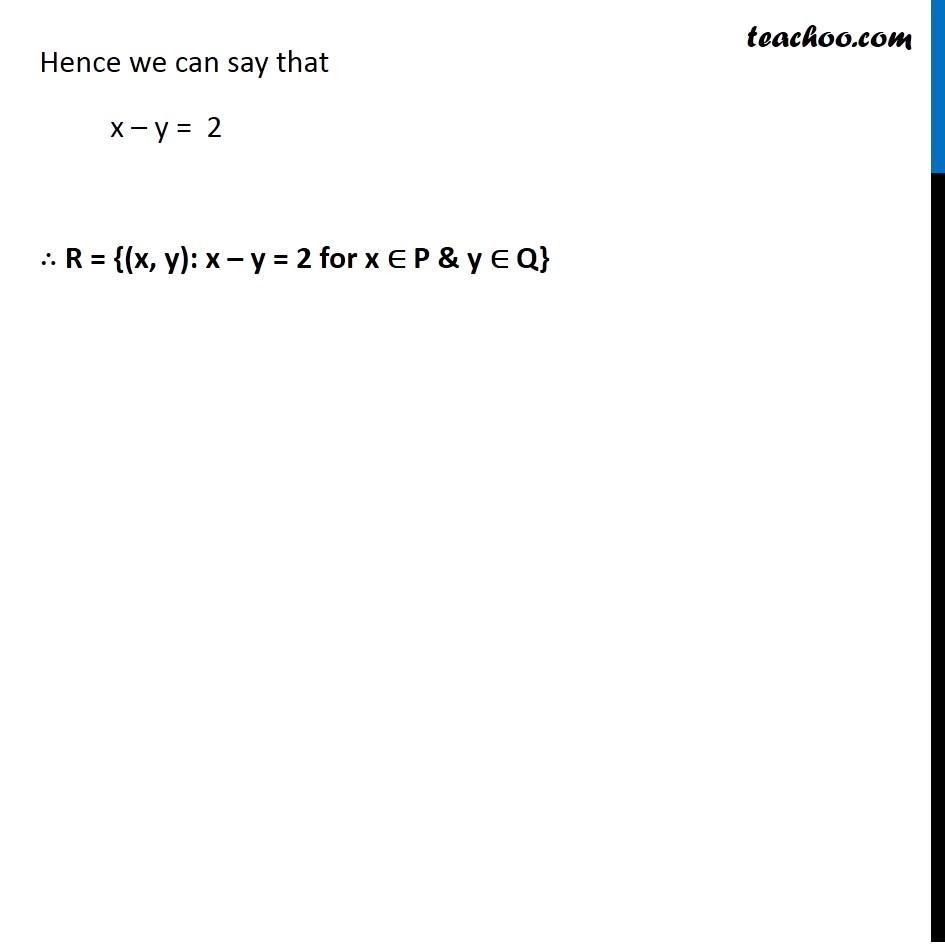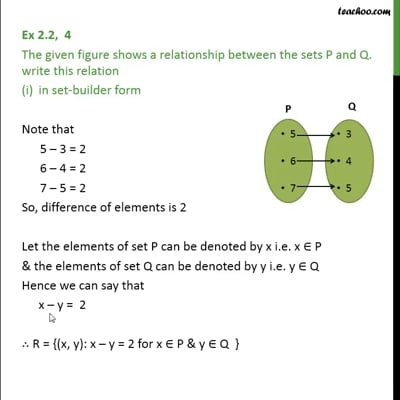This video is only available for Teachoo black users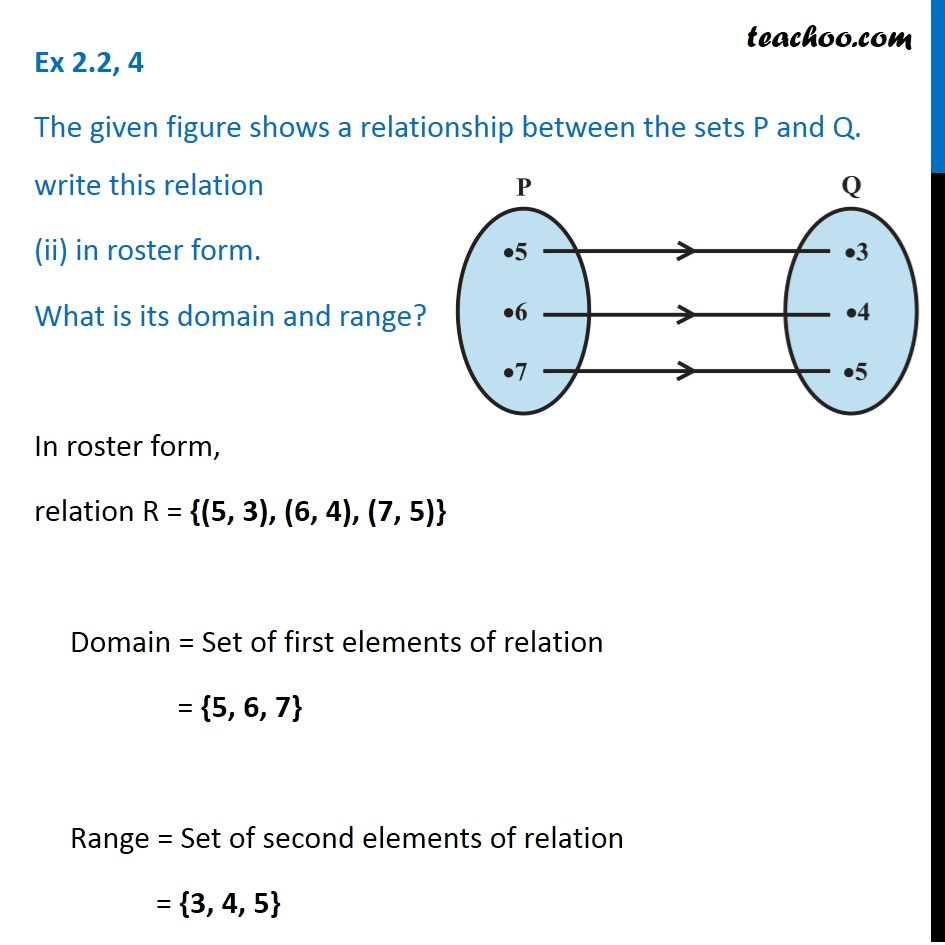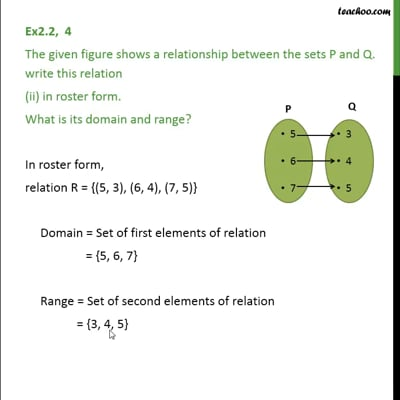This video is only available for Teachoo black users

Solve all your doubts with Teachoo Black (new monthly pack available now!)

### Transcript

Ex 2.2, 4 The given figure shows a relationship between the sets P and Q. write this relation in set-builder form Note that 5 – 3 = 2 6 – 4 = 2 7 – 5 = 2 So, difference of elements is 2 Let the elements of set P can be denoted by x i.e. x ∈ P & the elements of set Q can be denoted by y i.e. y ∈ Q Hence we can say that x – y = 2 ∴ R = {(x, y): x – y = 2 for x ∈ P & y ∈ Q} Ex 2.2, 4 The given figure shows a relationship between the sets P and Q. write this relation (ii) in roster form. What is its domain and range? In roster form, relation R = {(5, 3), (6, 4), (7, 5)} Domain = Set of first elements of relation = {5, 6, 7} Range = Set of second elements of relation = {3, 4, 5}